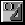# Converting Degrees to Radians

#### AutoCAD Visual LISP

Converting Degrees to Radians

You will now create a function to prevent you from repetitively typing an equation. It looks like this:

`(defun Degrees->Radians (numberOfDegrees) `
`(* pi (/ numberOfDegrees 180.0)))`

This function is called Degrees->Radians. The function name indicates its purpose.

Why do you need a function to convert angular measurements? Behind the scenes, AutoCAD® uses radian angular measurement to keep track of angles, whereas most people think in terms of degrees. This function in your toolkit allows you to think in degrees, and lets AutoLISP® convert those numbers to radians.

To test the utility function

1. Enter the following at the VLISP Console prompt:
`(defun Degrees->Radians (numberOfDegrees) `
`(* pi (/ numberOfDegrees 180.0)))`
2. Enter the following at the VLISP Console prompt:
`(degrees->radians 180)`

The function returns the number 3.14159. According to how this function works, 180 degrees is equivalent to 3.14159 radians.

To use this function within your program, simply copy the function definition from the Console window into your gpmain.lsp file. You can paste it anywhere in the file, as long as you do not paste it into the middle of an existing function.

To clean up your work, select the text you just pasted in, then choose the Format Selection button; VLISP will properly indent and format the code.Next, add some comments describing the function. When you have fully documented the function, your code should look something like this:

`;;;--------------------------------------------------------------;`
`;;;     Function: Degrees->Radians                               ;`
`;;;--------------------------------------------------------------;`
`;;; Description: This function converts a number representing an ;`
`;;;              angular measurement in degrees, into its radian ;`
`;;;              equivalent. There is no error checking on the   ;`
`;;;              numberOfDegrees parameter -- it is always       ;`
`;;;              expected to be a valid number.                  ;`
`;;;--------------------------------------------------------------;`
`(defun Degrees->Radians (numberOfDegrees)`
`  (* pi (/ numberOfDegrees 180.0))`
`)`